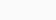# IIT JEE Solved Problem Examples for Limits, Continuity and Differentiability

IIT JEE Solved Problem Examples for Limits, Continuity and Differentiability includes Direct Substitution Method : If by direction substitution of the point in the given expression we get a finite number, then the number obtained is the limit of the number obtained is the limit. Rationlisation Method : This is a particularly used when either numerator or denominator or both involve expressions consisting of square roots as explained. Method of Evaluating Algebraic Limits when x ® ¥ : To evaluate this type of limits. Differentiability in a set (i) A function f (x) defined on an open interval (a, b) is
said to be differentiable or derivable in open interval (a,b) if it is differentiable at each point of (a, b).

## Download Solved Problem Examples for Limits, Continuity and Differentiability in PDF

### You may also like``` Related Pages: 1 2 3 4 5 6 7 8 9 10 ```
``` ```
``` ```
``` Post navigation ← Previous PostNext Post → ```
``` ```
``` Search Maxen Blogs Search for: इन्टरनेट से पैसा कैसे कमाए (विडियो देखें) (adsbygoogle=window.adsbygoogle||[]).push({}) Search Here :var aax_size='300x250';var aax_pubname='maxencomputer-21';var aax_src='302' CategoriesCCC (141)CCC Question Answer (105)CCC Study Material Notes (77)Question Papers (97)Courses at Maxen (20)CTET (10)CTET Question Answer (1)CTET UPTET Study Material (4)O Level (48)O Level Question Answer (17)O Level Solutions (5)O Level Study Material (27)TET (11)Andhra Pradesh TET (2)BTC (3)CG TET (1)Kerala TET (1)TN TET (4)Uncategorized (14)UPTET (12)UPTET Question Answer (3)UPTET Study Material (4)UPTET Study Material in English (2)UTET Study Material in Hindi (4)Recent Posts CCC Question Answer Sample Model Practice Sets in Hindi DOEACC CCC Sample Model Question Paper in Hindi CCC Question Answer Practice Paper Set in Hindi CCC DOEACC NIELIT Practice Sets in Hindi DOEACC NIELIT CCC Sample Model Question Paper DOEACC CCC Practice Question Answer in Hindi CCC Question Answer Sample Model Paper in Hindi 2 CCC Sample Paper in Hindi 1 CCC keyboard Shortcuts Abbreviations and Terminological Study CCC Sample Model Practice Demo Test Question Answer Paper 2020 (adsbygoogle=window.adsbygoogle||[]).push({})Recent Posts CCC Question Answer Sample Model Practice Sets in Hindi DOEACC CCC Sample Model Question Paper in Hindi CCC Question Answer Practice Paper Set in Hindi CCC DOEACC NIELIT Practice Sets in Hindi DOEACC NIELIT CCC Sample Model Question PaperRecent CommentsRishu on DOEACC NIELIT CCC Study Material Notes in Hindi and EnglishBest Computer Institute in Meerut… Lorem ipsum the rest paragraph will be here and this is the rest of the paragraph last line.```
``` var astra={"break_point":"768","isRtl":""} var localize={"ajaxurl":"https:\/\/maxen.pro\/wp-admin\/admin-ajax.php","nonce":"02e1458359","i18n":{"added":"Added ","compare":"Compare","loading":"Loading..."},"page_permalink":"https:\/\/maxen.pro\/iit-jee-solved-problem-examples-for-limits-continuity-and-differentiability\/"} var elementskit={resturl:'https://maxen.pro/wp-json/elementskit/v1/',} var elementorFrontendConfig={"environmentMode":{"edit":!1,"wpPreview":!1,"isScriptDebug":!1},"i18n":{"shareOnFacebook":"Share on Facebook","shareOnTwitter":"Share on Twitter","pinIt":"Pin it","download":"Download","downloadImage":"Download image","fullscreen":"Fullscreen","zoom":"Zoom","share":"Share","playVideo":"Play Video","previous":"Previous","next":"Next","close":"Close"},"is_rtl":!1,"breakpoints":{"xs":0,"sm":480,"md":768,"lg":1025,"xl":1440,"xxl":1600},"responsive":{"breakpoints":{"mobile":{"label":"Mobile","value":767,"default_value":767,"direction":"max","is_enabled":!0},"mobile_extra":{"label":"Mobile Extra","value":880,"default_value":880,"direction":"max","is_enabled":!1},"tablet":{"label":"Tablet","value":1024,"default_value":1024,"direction":"max","is_enabled":!0},"tablet_extra":{"label":"Tablet Extra","value":1200,"default_value":1200,"direction":"max","is_enabled":!1},"laptop":{"label":"Laptop","value":1366,"default_value":1366,"direction":"max","is_enabled":!1},"widescreen":{"label":"Widescreen","value":2400,"default_value":2400,"direction":"min","is_enabled":!1}}},"version":"3.5.6","is_static":!1,"experimentalFeatures":{"e_import_export":!0,"e_hidden_wordpress_widgets":!0,"landing-pages":!0,"elements-color-picker":!0,"favorite-widgets":!0,"admin-top-bar":!0},"urls":{"assets":"https:\/\/maxen.pro\/wp-content\/plugins\/elementor\/assets\/"},"settings":{"page":[],"editorPreferences":[]},"kit":{"global_image_lightbox":"yes","active_breakpoints":["viewport_mobile","viewport_tablet"],"lightbox_enable_counter":"yes","lightbox_enable_fullscreen":"yes","lightbox_enable_zoom":"yes","lightbox_enable_share":"yes","lightbox_title_src":"title","lightbox_description_src":"description"},"post":{"id":24409,"title":"IIT%20JEE%20Solved%20Problem%20Examples%20for%20Limits%2C%20Continuity%20and%20Differentiability%20%7C%20Maxen%20Computer%20Education","excerpt":"","featuredImage":!1}} var ekit_config={"ajaxurl":"https:\/\/maxen.pro\/wp-admin\/admin-ajax.php","nonce":"d16b123136"} var _wpUtilSettings={"ajax":{"url":"\/wp-admin\/admin-ajax.php"}} var wpformsElementorVars={"captcha_provider":"recaptcha","recaptcha_type":"v2"} /(trident|msie)/i.test(navigator.userAgent)&&document.getElementById&&window.addEventListener&&window.addEventListener("hashchange",function(){var t,e=location.hash.substring(1);/^[A-z0-9_-]+\$/.test(e)&&(t=document.getElementById(e))&&(/^(?:a|select|input|button|textarea)\$/i.test(t.tagName)||(t.tabIndex=-1),t.focus())},!1) _stq=window._stq||[];_stq.push(['view',{v:'ext',j:'1:10.7',blog:'202332692',post:'24409',tz:'5.5',srv:'maxen.pro'}]);_stq.push(['clickTrackerInit','202332692','24409']) !function(t,e){"object"==typeof exports&&"undefined"!=typeof module?module.exports=e():"function"==typeof define&&define.amd?define(e):(t="undefined"!=typeof globalThis?globalThis:t||self).LazyLoad=e()}(this,function(){"use strict";function e(){return(e=Object.assign||function(t){for(var e=1;e<arguments.length;e++){var n,a=arguments[e];for(n in a)Object.prototype.hasOwnProperty.call(a,n)&&(t[n]=a[n])}return t}).apply(this,arguments)}function i(t){return e({},it,t)}function o(t,e){var n,a="LazyLoad::Initialized",i=new t(e);try{n=new CustomEvent(a,{detail:{instance:i}})}catch(t){(n=document.createEvent("CustomEvent")).initCustomEvent(a,!1,!1,{instance:i})}window.dispatchEvent(n)}function l(t,e){return t.getAttribute(gt+e)}function c(t){return l(t,bt)}function s(t,e){return function(t,e,n){e=gt+e;null!==n?t.setAttribute(e,n):t.removeAttribute(e)}(t,bt,e)}function r(t){return s(t,null),0}function u(t){return null===c(t)}function d(t){return c(t)===vt}function f(t,e,n,a){t&&(void 0===a?void 0===n?t(e):t(e,n):t(e,n,a))}function _(t,e){nt?t.classList.add(e):t.className+=(t.className?" ":"")+e}function v(t,e){nt?t.classList.remove(e):t.className=t.className.replace(new RegExp("(^|\\s+)"+e+"(\\s+|\$)")," ").replace(/^\s+/,"").replace(/\s+\$/,"")}function g(t){return t.llTempImage}function b(t,e){!e||(e=e._observer)&&e.unobserve(t)}function p(t,e){t&&(t.loadingCount+=e)}function h(t,e){t&&(t.toLoadCount=e)}function n(t){for(var e,n=[],a=0;e=t.children[a];a+=1)"SOURCE"===e.tagName&&n.push(e);return n}function m(t,e){(t=t.parentNode)&&"PICTURE"===t.tagName&&n(t).forEach(e)}function a(t,e){n(t).forEach(e)}function E(t){return!!t[st]}function I(t){return t[st]}function y(t){return delete t[st]}function A(e,t){var n;E(e)||(n={},t.forEach(function(t){n[t]=e.getAttribute(t)}),e[st]=n)}function k(a,t){var i;E(a)&&(i=I(a),t.forEach(function(t){var e,n;e=a,(t=i[n=t])?e.setAttribute(n,t):e.removeAttribute(n)}))}function L(t,e,n){_(t,e.class_loading),s(t,ut),n&&(p(n,1),f(e.callback_loading,t,n))}function w(t,e,n){n&&t.setAttribute(e,n)}function x(t,e){w(t,ct,l(t,e.data_sizes)),w(t,rt,l(t,e.data_srcset)),w(t,ot,l(t,e.data_src))}function O(t,e,n){var a=l(t,e.data_bg_multi),i=l(t,e.data_bg_multi_hidpi);(a=at&&i?i:a)&&(t.style.backgroundImage=a,n=n,_(t=t,(e=e).class_applied),s(t,ft),n&&(e.unobserve_completed&&b(t,e),f(e.callback_applied,t,n)))}function N(t,e){!e||0<e.loadingCount||0<e.toLoadCount||f(t.callback_finish,e)}function C(t,e,n){t.addEventListener(e,n),t.llEvLisnrs[e]=n}function M(t){return!!t.llEvLisnrs}function z(t){if(M(t)){var e,n,a=t.llEvLisnrs;for(e in a){var i=a[e];n=e,i=i,t.removeEventListener(n,i)}delete t.llEvLisnrs}}function R(t,e,n){var a;delete t.llTempImage,p(n,-1),(a=n)&&--a.toLoadCount,v(t,e.class_loading),e.unobserve_completed&&b(t,n)}function T(o,r,c){var l=g(o)||o;M(l)||function(t,e,n){M(t)||(t.llEvLisnrs={});var a="VIDEO"===t.tagName?"loadeddata":"load";C(t,a,e),C(t,"error",n)}(l,function(t){var e,n,a,i;n=r,a=c,i=d(e=o),R(e,n,a),_(e,n.class_loaded),s(e,dt),f(n.callback_loaded,e,a),i||N(n,a),z(l)},function(t){var e,n,a,i;n=r,a=c,i=d(e=o),R(e,n,a),_(e,n.class_error),s(e,_t),f(n.callback_error,e,a),i||N(n,a),z(l)})}function G(t,e,n){var a,i,o,r,c;t.llTempImage=document.createElement("IMG"),T(t,e,n),E(c=t)||(c[st]={backgroundImage:c.style.backgroundImage}),o=n,r=l(a=t,(i=e).data_bg),c=l(a,i.data_bg_hidpi),(r=at&&c?c:r)&&(a.style.backgroundImage='url("'.concat(r,'")'),g(a).setAttribute(ot,r),L(a,i,o)),O(t,e,n)}function D(t,e,n){var a;T(t,e,n),a=e,e=n,(t=It[(n=t).tagName])&&(t(n,a),L(n,a,e))}function V(t,e,n){var a;a=t,(-1<yt.indexOf(a.tagName)?D:G)(t,e,n)}function F(t,e,n){var a;t.setAttribute("loading","lazy"),T(t,e,n),a=e,(e=It[(n=t).tagName])&&e(n,a),s(t,vt)}function j(t){t.removeAttribute(ot),t.removeAttribute(rt),t.removeAttribute(ct)}function P(t){m(t,function(t){k(t,Et)}),k(t,Et)}function S(t){var e;(e=At[t.tagName])?e(t):E(e=t)&&(t=I(e),e.style.backgroundImage=t.backgroundImage)}function U(t,e){var n;S(t),n=e,u(e=t)||d(e)||(v(e,n.class_entered),v(e,n.class_exited),v(e,n.class_applied),v(e,n.class_loading),v(e,n.class_loaded),v(e,n.class_error)),r(t),y(t)}function \$(t,e,n,a){var i;n.cancel_on_exit&&(c(t)!==ut||"IMG"===t.tagName&&(z(t),m(i=t,function(t){j(t)}),j(i),P(t),v(t,n.class_loading),p(a,-1),r(t),f(n.callback_cancel,t,e,a)))}function q(t,e,n,a){var i,o,r=(o=t,0<=pt.indexOf(c(o)));s(t,"entered"),_(t,n.class_entered),v(t,n.class_exited),i=t,o=a,n.unobserve_entered&&b(i,o),f(n.callback_enter,t,e,a),r||V(t,n,a)}function H(t){return t.use_native&&"loading"in HTMLImageElement.prototype}function B(t,i,o){t.forEach(function(t){return(a=t).isIntersecting||0<a.intersectionRatio?q(t.target,t,i,o):(e=t.target,n=t,a=i,t=o,void(u(e)||(_(e,a.class_exited),\$(e,n,a,t),f(a.callback_exit,e,n,t))));var e,n,a})}function J(e,n){var t;et&&!H(e)&&(n._observer=new IntersectionObserver(function(t){B(t,e,n)},{root:(t=e).container===document?null:t.container,rootMargin:t.thresholds||t.threshold+"px"}))}function K(t){return Array.prototype.slice.call(t)}function Q(t){return t.container.querySelectorAll(t.elements_selector)}function W(t){return c(t)===_t}function X(t,e){return e=t||Q(e),K(e).filter(u)}function Y(e,t){var n;(n=Q(e),K(n).filter(W)).forEach(function(t){v(t,e.class_error),r(t)}),t.update()}function t(t,e){var n,a,t=i(t);this._settings=t,this.loadingCount=0,J(t,this),n=t,a=this,Z&&window.addEventListener("online",function(){Y(n,a)}),this.update(e)}var Z="undefined"!=typeof window,tt=Z&&!("onscroll"in window)||"undefined"!=typeof navigator&&/(gle|ing|ro)bot|crawl|spider/i.test(navigator.userAgent),et=Z&&"IntersectionObserver"in window,nt=Z&&"classList"in document.createElement("p"),at=Z&&1<window.devicePixelRatio,it={elements_selector:".lazy",container:tt||Z?document:null,threshold:300,thresholds:null,data_src:"src",data_srcset:"srcset",data_sizes:"sizes",data_bg:"bg",data_bg_hidpi:"bg-hidpi",data_bg_multi:"bg-multi",data_bg_multi_hidpi:"bg-multi-hidpi",data_poster:"poster",class_applied:"applied",class_loading:"litespeed-loading",class_loaded:"litespeed-loaded",class_error:"error",class_entered:"entered",class_exited:"exited",unobserve_completed:!0,unobserve_entered:!1,cancel_on_exit:!0,callback_enter:null,callback_exit:null,callback_applied:null,callback_loading:null,callback_loaded:null,callback_error:null,callback_finish:null,callback_cancel:null,use_native:!1},ot="src",rt="srcset",ct="sizes",lt="poster",st="llOriginalAttrs",ut="loading",dt="loaded",ft="applied",_t="error",vt="native",gt="data-",bt="ll-status",pt=[ut,dt,ft,_t],ht=[ot],mt=[ot,lt],Et=[ot,rt,ct],It={IMG:function(t,e){m(t,function(t){A(t,Et),x(t,e)}),A(t,Et),x(t,e)},IFRAME:function(t,e){A(t,ht),w(t,ot,l(t,e.data_src))},VIDEO:function(t,e){a(t,function(t){A(t,ht),w(t,ot,l(t,e.data_src))}),A(t,mt),w(t,lt,l(t,e.data_poster)),w(t,ot,l(t,e.data_src)),t.load()}},yt=["IMG","IFRAME","VIDEO"],At={IMG:P,IFRAME:function(t){k(t,ht)},VIDEO:function(t){a(t,function(t){k(t,ht)}),k(t,mt),t.load()}},kt=["IMG","IFRAME","VIDEO"];return t.prototype={update:function(t){var e,n,a,i=this._settings,o=X(t,i);{if(h(this,o.length),!tt&&et)return H(i)?(e=i,n=this,o.forEach(function(t){-1!==kt.indexOf(t.tagName)&&F(t,e,n)}),void h(n,0)):(t=this._observer,i=o,t.disconnect(),a=t,void i.forEach(function(t){a.observe(t)}));this.loadAll(o)}},destroy:function(){this._observer&&this._observer.disconnect(),Q(this._settings).forEach(function(t){y(t)}),delete this._observer,delete this._settings,delete this.loadingCount,delete this.toLoadCount},loadAll:function(t){var e=this,n=this._settings;X(t,n).forEach(function(t){b(t,e),V(t,n,e)})},restoreAll:function(){var e=this._settings;Q(e).forEach(function(t){U(t,e)})}},t.load=function(t,e){e=i(e);V(t,e)},t.resetStatus=function(t){r(t)},Z&&function(t,e){if(e)if(e.length)for(var n,a=0;n=e[a];a+=1)o(t,n);else o(t,e)}(t,window.lazyLoadOptions),t});!function(e,t){"use strict";function a(){t.body.classList.add("litespeed_lazyloaded")}function n(){console.log("[LiteSpeed] Start Lazy Load Images"),d=new LazyLoad({elements_selector:"[data-lazyloaded]",callback_finish:a}),o=function(){d.update()},e.MutationObserver&&new MutationObserver(o).observe(t.documentElement,{childList:!0,subtree:!0,attributes:!0})}var d,o;e.addEventListener?e.addEventListener("load",n,!1):e.attachEvent("onload",n)}(window,document);var litespeed_vary=document.cookie.replace(/(?:(?:^|.*;\s*)_lscache_vary\s*\=\s*([^;]*).*\$)|^.*\$/,"");litespeed_vary||fetch("/wp-content/plugins/litespeed-cache/guest.vary.php",{method:"POST",cache:"no-cache",redirect:"follow"}).then(e=>e.json()).then(e=>{console.log(e),e.hasOwnProperty("reload")&&"yes"==e.reload&&(sessionStorage.setItem("litespeed_docref",document.referrer),window.location.reload(!0))});const litespeed_ui_events=["mouseover","click","keydown","wheel","touchmove","touchstart"];var urlCreator=window.URL||window.webkitURL;function litespeed_load_delayed_js_force(){console.log("[LiteSpeed] Start Load JS Delayed"),litespeed_ui_events.forEach(e=>{window.removeEventListener(e,litespeed_load_delayed_js_force,{passive:!0})}),document.querySelectorAll("iframe[data-litespeed-src]").forEach(e=>{e.setAttribute("src",e.getAttribute("data-litespeed-src"))}),"loading"==document.readyState?window.addEventListener("DOMContentLoaded",litespeed_load_delayed_js):litespeed_load_delayed_js()}litespeed_ui_events.forEach(e=>{window.addEventListener(e,litespeed_load_delayed_js_force,{passive:!0})});async function litespeed_load_delayed_js(){let t=[];for(var d in document.querySelectorAll('script[type="litespeed/javascript"]').forEach(e=>{t.push(e)}),t)await new Promise(e=>litespeed_load_one(t[d],e));document.dispatchEvent(new Event("DOMContentLiteSpeedLoaded")),window.dispatchEvent(new Event("DOMContentLiteSpeedLoaded"))}function litespeed_load_one(t,e){console.log("[LiteSpeed] Load ",t);var d=document.createElement("script");d.addEventListener("load",e),d.addEventListener("error",e),t.getAttributeNames().forEach(e=>{"type"!=e&&d.setAttribute("data-src"==e?"src":e,t.getAttribute(e))});let a=!(d.type="text/javascript");!d.src&&t.textContent&&(d.src=litespeed_inline2src(t.textContent),a=!0),t.after(d),t.remove(),a&&e()}function litespeed_inline2src(t){try{var d=urlCreator.createObjectURL(new Blob([t.replace(/^(?:<!--)?(.*?)(?:-->)?\$/gm,"\$1")],{type:"text/javascript"}))}catch(e){d="data:text/javascript;base64,"+btoa(t.replace(/^(?:<!--)?(.*?)(?:-->)?\$/gm,"\$1"))}return d} ```